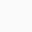# A body moving with constant acceleration | travels the distance 3 metre and 8 metre respectively in 1 second and 2 second | calculate the initial velocity and the acceleration of body | Sum Motion in one Dimension – Physics class 9

Problem :     A body moving with constant acceleration travels the distance 3 metre and 8 metre respectively in one second 2 second. Calculate the 1) initial velocity and 2) the acceleration of body.

Data given:
Distance(s1) = 3m
Time taken (t1) to travel 3 m =1 second
Distance(s2) = 8m
Time taken (t2) to travel 8 m =2 second

Initial velocity (u)= ?
Acceleration (a) = ?

Case 1:

We know

s = ut + 1/2 a. t2
Or s1 = ut1 + 1/2 a. t12
or 3 = u . 1 + 1/2 .a . 12
or 3 = u + a/2 ——-Eqn. 1

Case 2:

s2 = ut2 + 1/2 a. t22
or 8 = u . 2 + 1/2 .a . 22
or 8 = 2u + 2a
or 8/2 = (2u + 2a)/2
or 4 = u + a ——-Eqn. 2
Subracting Eqn. 1 from Eqn. 2 (Eqn. 2 – Eqn. 1) We have:
4 – 3 = (u + a) – (u + a/2)
Or 1 = u + a – u – a/2
Or 1 = a – a/2
Or 1 = (2a – a)/2
Or 1 = a /2
Or a = 2 m/s2
Putting the value of a in Eqn. 1 we have:
3 = u + 2/2
3 = u + 1
Or u = 3 – 1
Or u = 2 m/s
∴ Initial velocity (u)= 2 m/s
Acceleration (a) = 2 m/s2 Answer

##### 2 thoughts on “A body moving with constant acceleration | travels the distance 3 metre and 8 metre respectively in 1 second and 2 second | calculate the initial velocity and the acceleration of body | Sum Motion in one Dimension – Physics class 9”
1.Unknown says:

From which book is this question covered

2.Unknown says: